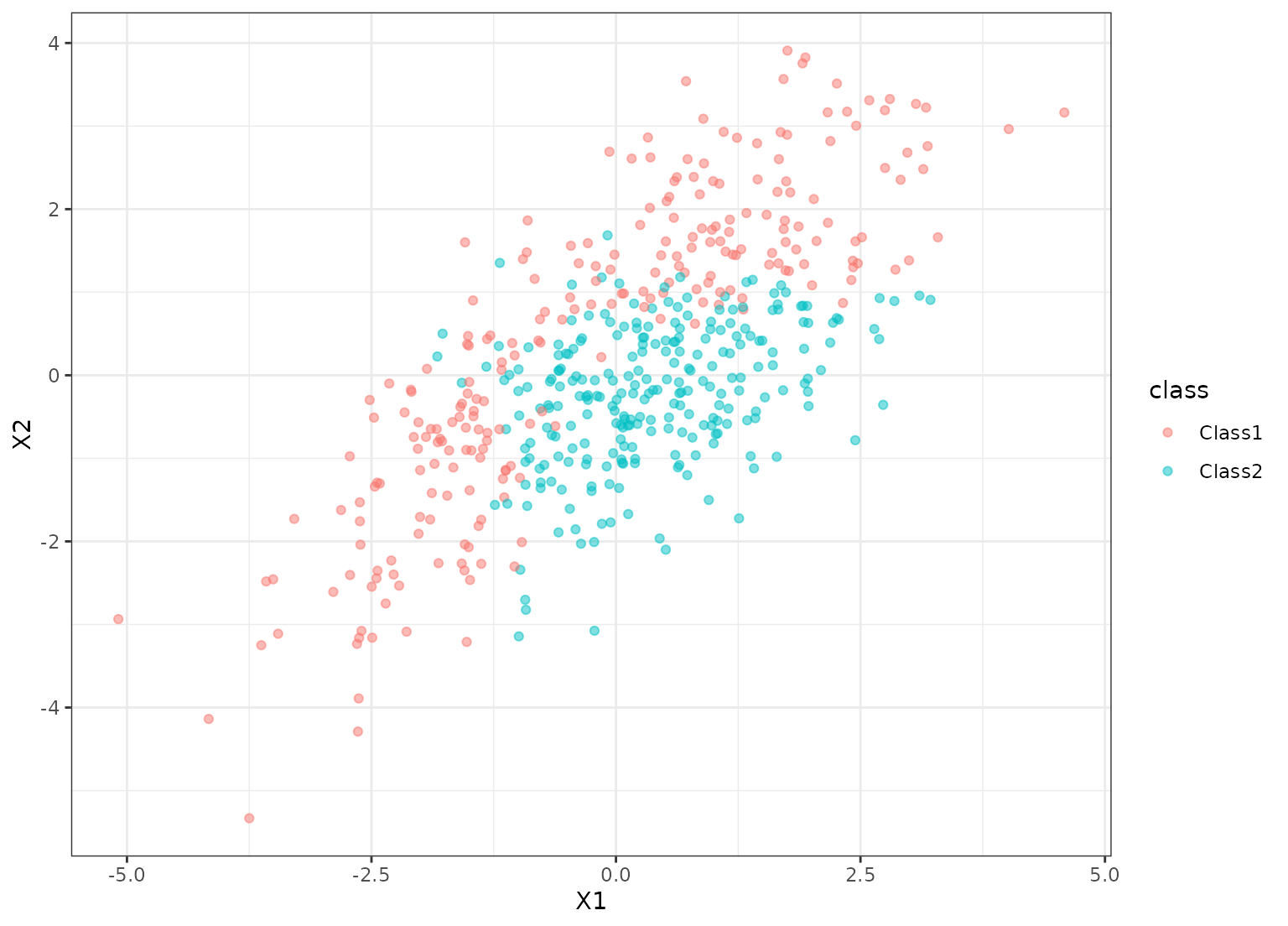Parabolic class boundary data

parabolic

a data frame

## Details

These data were simulated. There are two correlated predictors and two classes in the factor outcome.

## Examples

data(parabolic)

library(ggplot2)
ggplot(parabolic, aes(x = X1, y = X2, col = class)) +
geom_point(alpha = .5) +
theme_bw()